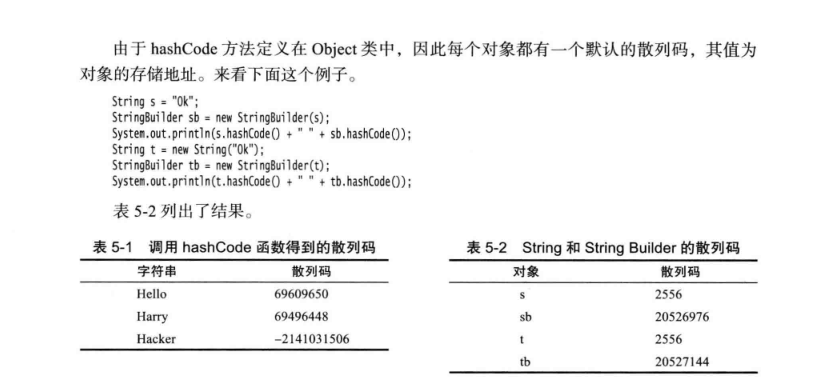# 前言

package main

import "fmt"

func main() {
var a int= 20   /* 声明实际变量 */
var ip *int        /* 声明指针变量 */

ip = &a  /* 指针变量的存储地址 */
fmt.Printf("a 变量的地址是: %x\n", &a  )

/* 指针变量的存储地址 */
fmt.Printf("ip 变量储存的指针地址: %x\n", ip )
/* 使用指针访问值 */
fmt.Printf("*ip 变量的值: %d\n", *ip )
}# ==和equals

//Indicates whether some other object is "equal to" this one.
public boolean equals(Object obj) {
return (this == obj);
}


Tips：这里额外解释个疑惑

public boolean equals(Object anObject) {
if (this == anObject) {
return true;
}
if (anObject instanceof String) {
String anotherString = (String)anObject;
int n = value.length;
if (n == anotherString.value.length) {
char v1[] = value;
char v2[] = anotherString.value;
int i = 0;
while (n-- != 0) {
if (v1[i] != v2[i])
return false;
i++;
}
return true;
}
}
return false;
}


JDK 中不止 String 类重写了equals 方法，还有数据类型 Integer，Long，Double，Float等基本也都重写了 equals 方法。所以我们在代码中用 Long 或者 Integer 做业务参数的时候，如果要比较它们是否相等，记得需要使用 equals 方法，而不要使用 ==

# Object中的HashCode

equals 方法能比较两个对象的内容是否相等，因此可以用来查找某个对象是否在集合容器中，通常大致就是逐一去取集合中的每个对象元素与需要查询的对象进行equals比较，当发现某个元素与要查找的对象进行equals方法比较的结果相等时，则停止继续查找并返回肯定的信息，否则，返回否定的信息。

/**
* Returns a hash code value for the object. This method is
* supported for the benefit of hash tables such as those provided by
* ...
* As much as is reasonably practical, the hashCode method defined by
* class {@code Object} does return distinct integers for distinct
* objects. (This is typically implemented by converting the internal
* address of the object into an integer, but this implementation
* technique is not required by the
*
* @return  a hash code value for this object.
* @see     java.lang.Object#equals(java.lang.Object)
* @see     java.lang.System#identityHashCode
*/
public native int hashCode();


static JNINativeMethod methods[] = {
{"hashCode",    "()I",                    (void *)&JVM_IHashCode},
{"wait",        "(J)V",                   (void *)&JVM_MonitorWait},
{"notify",      "()V",                    (void *)&JVM_MonitorNotify},
{"notifyAll",   "()V",                    (void *)&JVM_MonitorNotifyAll},
{"clone",       "()Ljava/lang/Object;",   (void *)&JVM_Clone},
};


JVM_IHashCode方法实现在 jvm.cpp中的定义为：

JVM_ENTRY(jint, JVM_IHashCode(JNIEnv* env, jobject handle))
JVMWrapper("JVM_IHashCode");
// as implemented in the classic virtual machine; return 0 if object is NULL
return handle == NULL ? 0 : ObjectSynchronizer::FastHashCode (THREAD, JNIHandles::resolve_non_null(handle)) ;
JVM_END


intptr_t ObjectSynchronizer::FastHashCode (Thread * Self, oop obj) {
if (UseBiasedLocking) {

......

// Inflate the monitor to set hash code
monitor = ObjectSynchronizer::inflate(Self, obj);
assert (mark->is_neutral(), "invariant") ;
hash = mark->hash();
if (hash == 0) {
hash = get_next_hash(Self, obj);
temp = mark->copy_set_hash(hash); // merge hash code into header
assert (temp->is_neutral(), "invariant") ;
test = (markOop) Atomic::cmpxchg_ptr(temp, monitor, mark);
if (test != mark) {
// The only update to the header in the monitor (outside GC)
// is install the hash code. If someone add new usage of
hash = test->hash();
assert (test->is_neutral(), "invariant") ;
assert (hash != 0, "Trivial unexpected object/monitor header usage.");
}
}
// We finally get the hash
return hash;
}


static inline intptr_t get_next_hash(Thread * Self, oop obj) {
intptr_t value = 0 ;
if (hashCode == 0) {
// This form uses an unguarded global Park-Miller RNG,
// so it's possible for two threads to race and generate the same RNG.
// On MP system we'll have lots of RW access to a global, so the
// mechanism induces lots of coherency traffic.
value = os::random() ;
} else
if (hashCode == 1) {
// This variation has the property of being stable (idempotent)
// between STW operations.  This can be useful in some of the 1-0
// synchronization schemes.
intptr_t addrBits = cast_from_oop<intptr_t>(obj) >> 3 ;
} else
if (hashCode == 2) {
value = 1 ;            // for sensitivity testing
} else
if (hashCode == 3) {
value = ++GVars.hcSequence ;
} else
if (hashCode == 4) {
value = cast_from_oop<intptr_t>(obj) ;
} else {
// Marsaglia's xor-shift scheme with thread-specific state
// This is probably the best overall implementation -- we'll
// likely make this the default in future releases.
unsigned t = Self->_hashStateX ;
t ^= (t << 11) ;
Self->_hashStateX = Self->_hashStateY ;
Self->_hashStateY = Self->_hashStateZ ;
Self->_hashStateZ = Self->_hashStateW ;
unsigned v = Self->_hashStateW ;
v = (v ^ (v >> 19)) ^ (t ^ (t >> 8)) ;
Self->_hashStateW = v ;
value = v ;
}

if (value == 0) value = 0xBAD ;
assert (value != markOopDesc::no_hash, "invariant") ;
TEVENT (hashCode: GENERATE) ;
return value;
}


get_next_hash的方法中我们可以看到，如果从0开始算的话，这里提供了6种计算 hash 值的方案，有自增序列，随机数，关联内存地址等多种方式，其中官方默认的是最后一种，即随机数生成。可以看出 hashCode 也许和内存地址有关系，但不是直接代表内存地址的，具体需要看虚拟机版本和设置。

# equals和hashCode

equals 和 hashCode 都是 Object 类拥有的方法，包括 Object 类中的 toString 方法打印的内容也包含 hashCode 的无符号十六进制值。

public String toString() {
return getClass().getName() + "@" + Integer.toHexString(hashCode());
}


• 在应用程序的执行期间，只要对象的 equals 方法的比较操作所用到的信息没有被修改，那么对同一个对象的多次调用，hashCode 方法都必须始终返回同一个值。

• 如果两个对象根据 equals 方法比较是相等的，那么调用这两个对象中的 hashCode 方法都必须产生同样的整数结果。

• 如果两个对象根据 equals 方法比较是不相等的，那么调用者两个对象中的 hashCode 方法，则不一定要求 hashCode 方法必须产生不同的结果。但是给不相等的对象产生不同的整数散列值，是有可能提高散列表（hash table）的性能。

public class Student {

private String name;

private String gender;

public Student(String name, String gender) {
this.name = name;
this.gender = gender;
}

//省略 Setter，Gettter

@Override
public boolean equals(Object anObject) {
if (this == anObject) {
return true;
}
if (anObject instanceof Student) {
Student anotherStudent = (Student) anObject;

if (this.getName() == anotherStudent.getName()
|| this.getGender() == anotherStudent.getGender())
return true;
}
return false;
}
}


public static void main(String[] args) {

Student student1 = new Student("小明", "male");
Student student2 = new Student("小明", "male");

System.out.println("equals结果：" + student1.equals(student2));
System.out.println("对象1的散列值：" + student1.hashCode() + "，对象2的散列值：" + student2.hashCode());
}


equals结果：true



public static void main(String[] args) {

Student student1 = new Student("小明", "male");
Student student2 = new Student("小明", "male");

HashMap<Student, String> hashMap = new HashMap<>();
hashMap.put(student1, "小明");

String value = hashMap.get(student2);
System.out.println(value);
}


null


public static native int identityHashCode(Object x);


# 总结

posted @ 2019-08-12 09:11  JaJian  阅读(1820)  评论(1编辑  收藏